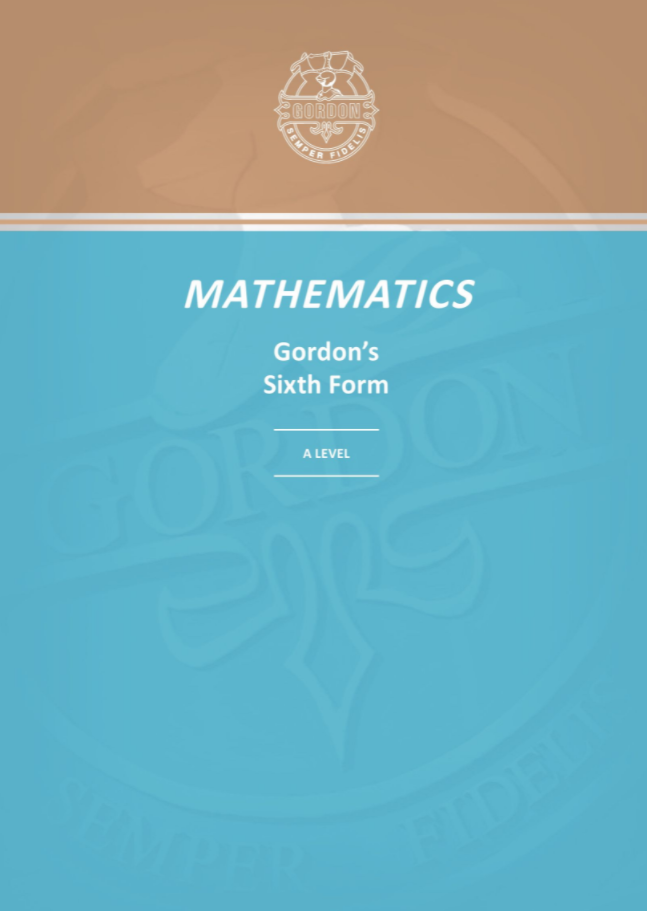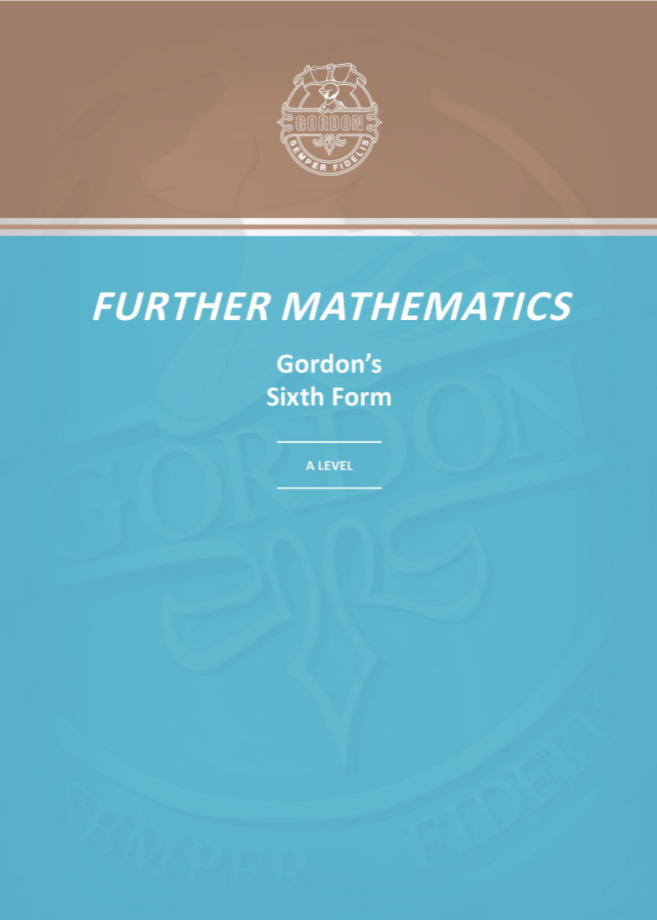# Mathematics

KS3
KS4
KS5 (Sixth Form)

## Summary

The Key Stage 3 Mathematics course follows the National Curriculum requirements, covering topics on number, algebra, ratio, proportion and rates of change, geometry and measures, probability and statistics. The course has two key objectives. Firstly, we want to build and foster our students' interest in, and enjoyment of, learning the subject of Mathematics. We achieve this by providing engaging lessons, which include a variety of tasks from group work to learning games to opportunities for independent inquiry. Secondly, we want our students to be able to excel at Key Stages 4 and 5. We achieve this by focussing on two key aspects of learning; basic numeracy skills and problem-solving. Numeracy booklets are used as starters each week and give students the opportunity to embed the key number concepts before entering Key Stage 4. Problem-solving is included in every topic and students are encouraged to complete the “Problem of the Week” outside the classroom.

## Summary

The Key Stage 4 Mathematics course (Edexcel syllabus)  is now more substantial and challenging with greater emphasis on problem-solving. In lessons, students will work on developing fluency and improving their skills of applying their knowledge in a variety of contexts. Students will sit three equally-weighted papers at either Foundation or Higher Tier . The majority of our students will sit the Higher Tier papers, which cover content at grades 4 to 9. Students will study these topics in greater depth and will also have the opportunity of studying more advanced techniques, which will prepare students for further study at A Level.

## Course details

The new GCSE Mathematics Specification is designed for two ability levels: Foundation and Higher.

Foundation Tier covers grades 1 to 5 and focuses on the key skills: algebra, ratio, proportion and rates of change, geometry and measures, probability and statistics

Higher Tier prepares pupils for AS/A Level mathematics and covers grades 4 to 9. This covers topics to a greater depth and includes more advanced techniques.

TYPICAL ACTIVITIES

The aims and objectives of the course are to enable students to:

• Develop fluent knowledge, skills and understanding of mathematical methods and concepts.
• Acquire, select and apply mathematical techniques to solve problems.
• Reason mathematically, make deductions and inferences and draw conclusions.
• Comprehend, interpret and communicate mathematical information in a variety of forms appropriate to the information and concepts.

## Summary

Mathematics

Students studying AS and A level Mathematics at KS5 (Edexcel Syllabus) will study both Pure Mathematics and Applied Mathematics. The course is designed to encourage students to develop their understanding of mathematics and mathematical processes, to develop their ability to reason logically, to generalise and to construct mathematical proofs and to use mathematics as an effective means of communication.

Pure Mathematics is concerned with algebra and functions, coordinate geometry, sequences and series, trigonometry, exponentials and logarithms, calculus, proof and vectors. Applied Mathematics is divided into two sections; Statistics and Mechanics. Statistics is the study of data presentation and interpretation, probability, statistical distributions and hypothesis testing. Mechanics applies mathematics to kinematics, forces, Newton’s laws and moments.

Further Mathematics

We also offer Further Mathematics to our most able students. Students will complete an A level in Mathematics in Year 12 and then complete an A level in Further Mathematics in Year 13. The course is designed to both broaden and deepen the work covered in A Level Mathematics and should be for those students who are looking to study a mathematics-rich degree at university.

Students will study the compulsory elements of Further Pure Mathematics, which will involve studying proof, complex numbers, matrices, polar coordinates, hyperbolic functions, differential equations and further work on algebra and functions, calculus and vectors. In addition to this, students will study two optional elements, which will be selected from Decision Mathematics, Further Statistics and Further Mechanics.

## Course details

Mathematics

AS Level Mathematics
Elements of Pure Mathematics and Applied Mathematics:
Students will study elements of Pure Mathematics (proof, algebra and functions, coordinate geometry, sequences and series, trigonometry, exponentials and logarithms, differentiation, integration, and vectors), elements of Statistics (statistical sampling, data presentation and interpretation, probability, statistical distributions, statistical hypothesis testing) and elements of Mechanics (quantities and units, kinematics, forces and Newton’s laws)

A Level Mathematics

Pure Mathematics and Applied Mathematics: Students will study elements of Pure Mathematics (proof, algebra and functions, coordinate geometry, sequences and series, trigonometry, exponentials and logarithms, differentiation, integration, numerical methods and vectors), elements of Statistics (statistical sampling, data presentation and interpretation, probability, statistical distributions, statistical hypothesis testing) and elements of Mechanics (quantities and units, kinematics, forces, Newton’s laws and moments)

All students completing the A Level in Mathematics must sit three exams at the end of Year 13, regardless of whether they were tested at the end of Year 12

Further Mathematics

A Level Mathematics:

Pure Mathematics and Applied Mathematics Year 12: Students will study elements of Pure Mathematics (proof, algebra and functions, coordinate geometry, sequences and series, trigonometry, exponentials and logarithms, differentiation, integration, numerical methods and vectors), elements of Statistics (statistical sampling, data presentation and interpretation, probability, statistical distributions, statistical hypothesis testing) and elements of Mechanics (quantities and units, kinematics, forces, Newton’s laws and moments)

All students completing the A Level in Mathematics must sit three exams at the end of Year 12, all at A Level standard.

A Level Further Mathematics:

Pure Mathematics and Applied Mathematics Year 13: Students will study the compulsory elements of Further Pure Mathematics (proof, complex numbers, matrices, further algebra and functions, further calculus, further vectors, polar coordinates, hyperbolic functions, differential equations). In addition to this, students will study two optional elements, which will be selected from Decision Mathematics, Further Statistics and Further Mechanics

## Entry Criteria

Maths: GCSE grade 7 in MathematicsFurther Maths: GCSE grade 7 Mathematics and must study AS Level Mathematics